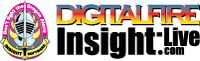•The secret to cool bodies and glazes is a lot of testing.
•The secret to know what to test is material and chemistry knowledge.
•The secret to learning from testing is documentation.
•The place to test, do the chemistry and document is an account at https://insight-live.com
•The place to get the knowledge is https://digitalfire.com

Sign-up at https://insight-live.com today.

# Whitfield 202 Fireclay

 Oxide Analysis Formula CaO 0.32% 0.049 MgO 0.27% 0.057 K2O 0.57% 0.052 Na2O 0.09% 0.012 P2O5 0.04% 0.002 TiO2 2.26% 0.242 Al2O3 11.90% 1.000 SiO2 77.00% 10.982 Fe2O3 1.57% 0.084 SO4 5.50 Oxide Weight 805.89 Formula Weight 852.79

## XML for Import into INSIGHT

<?xml version="1.0" encoding="UTF-8"?> <material name="Whitfield 202 Fireclay" descrip="" searchkey="" loi="0.00" casnumber=""> <oxides> <oxide symbol="CaO" name="Calcium Oxide, Calcia" status="" percent="0.320" tolerance=""/> <oxide symbol="MgO" name="Magnesium Oxide, Magnesia" status="" percent="0.270" tolerance=""/> <oxide symbol="K2O" name="Potassium Oxide" status="" percent="0.570" tolerance=""/> <oxide symbol="Na2O" name="Sodium Oxide, Soda" status="" percent="0.090" tolerance=""/> <oxide symbol="P2O5" name="Phosphorus Pentoxide" status="" percent="0.040" tolerance=""/> <oxide symbol="TiO2" name="Titanium Dioxide, Titania" status="" percent="2.260" tolerance=""/> <oxide symbol="Al2O3" name="Aluminum Oxide, Alumina" status="" percent="11.900" tolerance=""/> <oxide symbol="SiO2" name="Silicon Dioxide, Silica" status="" percent="77.000" tolerance=""/> <oxide symbol="Fe2O3" name="Iron Oxide, Ferric Oxide" status="" percent="1.570" tolerance=""/> </oxides> <volatiles> <volatile symbol="SO4" name="Sulfate" percent="5.500" tolerance=""/> </volatiles> </material>